• ## pythonTopK算法

千次阅读 2019-09-20 10:42:43
TopK算法 寻找数组中的最小的k个数，也叫topk问题。 该算法要解决的问题是：在线性时间内找到一个无序序列中第kk大的数。 如：输入n个整数，找出其中最小的K个数。例如输入4,5,1,6,2,7,3,8这8个数字，则最小的4个...
TopK算法
寻找数组中的最小的k个数，也叫topk问题。
该算法要解决的问题是：在线性时间内找到一个无序序列中第 kk 大的数。
如：输入n个整数，找出其中最小的K个数。例如输入4,5,1,6,2,7,3,8这8个数字，则最小的4个数字是1,2,3,4,。
思路：
快速排序的 partition() 方法，会返回一个整数 j 使得 a[l…j-1] 小于等于 a[j]，且 a[j+1…h] 大于等于 a[j]，此时 a[j] 就是数组的第 j 大元素。可以利用这个特性找出数组的第 K 个元素，这种找第 K 个元素的算法称为快速选择算法。

# -*- coding: gbk -*-
def partition(seq):
pi, seq = seq, seq[1:]                 # 选取并移除主元
lo = [x for x in seq if x <= pi]#选出小于第一个数的所有元素
hi = [x for x in seq if x > pi]##选出大于第一个数的所有元素
return lo, pi, hi

def select(seq, k):
lo, pi, hi = partition(seq)
m = len(lo)#小于第一个数的元素有几个
if m == k: return pi
if m < k: return select(hi, k-m-1)
return select(lo, k)

if __name__ == '__main__':
seq=(1,2,3,4,5)
print(partition(seq))
print(select(seq,3))

展开全文• 主要介绍了pythontopk算法实例，具有很好的参考价值，希望对大家有所帮助。一起跟随小编过来看看吧
• 现在又n个数，设计算法得到前k大的数 (k<n) 解决思路 排序后切片O(nlogn) 排序LowB三人组 O(mn) m取10个 1 取列表前k个元素建立一个小根堆。堆顶就是目前第k大的数 依次向后遍历原列表，对于列表中的元素，...
现在又n个数，设计算法得到前k大的数 (k<n)
解决思路
排序后切片O(nlogn)排序LowB三人组 O(kn) k取100个堆排序思路 O(nlogk) k 是27
1
取列表前k个元素建立一个小根堆。堆顶就是目前第k大的数  依次向后遍历原列表，对于列表中的元素，如果小于堆顶，则忽略该元素；如果大于堆顶，则将堆顶更换为该元素，并且 对堆进行一次调整  遍历列表所有元素后，倒序弹出堆顶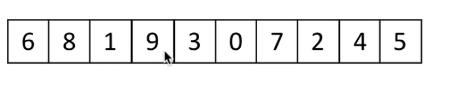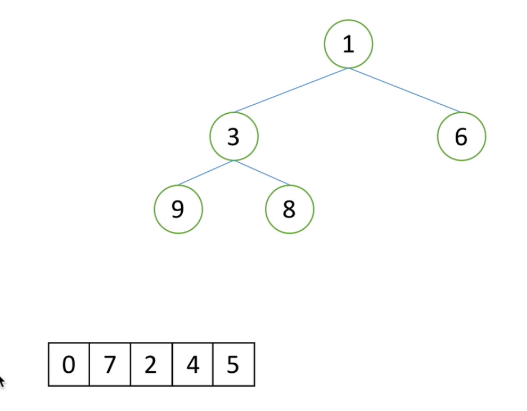1在堆里面最小的
小的放弃 ，大的替换在比较
要是7，7就把1替换掉，3和7替换，3上去，7下来了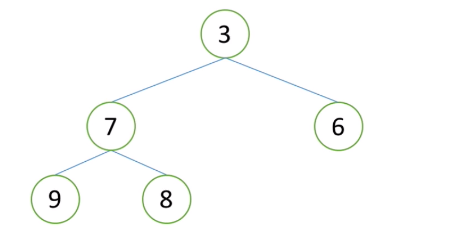4、5都别3大，替换掉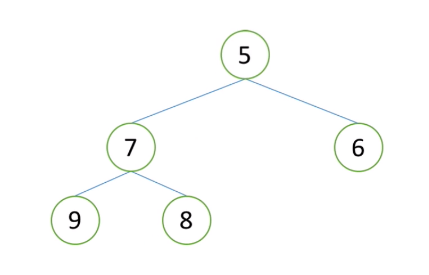#li 列表
# low: 堆的根节点位置 第一个元素
# higth 堆的指向最后一个元素的位置
# return
#i->指当前一层 和下一个层
#i 是市长
#i要是大于higth 退出了
#n-1 就是是整个堆元素最后一个下标

def sift(li,low,hight):
i = low
#找孩子 左孩子
j = 2 * i +1
tmp = li[low] #把堆顶存起来
while  j <= hight: #只要j位置有数
#右孩子要右，右孩子比较大 并且右孩子大于左孩子     右孩子不越界 j+1 <=hight
if j+1 <=hight  and li[j+1] < li[j]:
#j 指向右孩子  这个指的是两个孩子更大的数
j = j + 1
if li[j] < tmp:  #目前要是,tmp大就放过去,还是j大放上面去
li[i] = li[j]
i = j       #可以交换了    现在j等于新的i     i等于原来的j
j = 2 * i + 1
else :  #tmp 更大,把tmp放到i的位置上  假如堆点是6
li[i] = tmp  # 6放到原来8的
break
else: #就是2没法和别的比了,就放到该去的地方去
li[i] = tmp  #把tmp放到叶子节点上去

#最后非叶子节点
#n的最后一个下标是n-1,找他的父亲就孩子,(n-1-1)/2
#孩子下标是 n
#孩子找父亲 (n-1)/2 里面的n的最后一个下标是n-1 所有(n-1-1)/2 -> (n-2)/2

def topk(li,k):
heap = li[0:k]
for i in range((k-2)//2,-1,-1):
sift(heap,i,k-1)

#1.建堆
for i in range(k,len(li)-1):
if li[i] > heap:
heap = li[i]
sift(heap,0,k-1)
#print(heap)

#2. 遍历
for i in range(k-1,-1,-1):
heap, heap[i] = heap[i], heap
sift(heap,0,i -1)
return heap

import random
li = list(range(1000))
random.shuffle(li)

print(topk(li,10))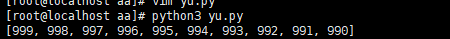展开全文• ## topk问题python解

千次阅读 2018-11-07 14:07:49
题目来源：Leetcode 215 数组中第K个最大的元素 题目描述： 在未排序的数组中找到第 k 个最大的元素。请注意，你需要找的是数组排序后...复杂度为O(nlogn)，可以直接调用python的排序函数做（用时52ms）: class ...
题目来源：Leetcode 215 数组中第K个最大的元素
题目描述：
在未排序的数组中找到第 k 个最大的元素。请注意，你需要找的是数组排序后的第 k 个最大的元素，而不是第 k 个不同的元素。
示例 1:
输入: [3,2,1,5,6,4] 和 k = 2
输出: 5
1.排序方法
复杂度为O(nlogn)，可以直接调用python的排序函数做（用时52ms）:
class Solution:
def findKthLargest(self, nums, k):
"""
:type nums: List[int]
:type k: int
:rtype: int
"""
nums.sort()
return nums[-k]

自己手写快排(用时284ms,咋这么慢。。。)：
class Solution:
def findKthLargest(self, nums, k):
"""
:type nums: List[int]
:type k: int
:rtype: int
"""
import random
self.quickSort(nums, 0, len(nums) - 1)
return nums[-k]

def partation(self, nums, l, r):
"""随机partation"""
change_index = random.randint(l, r)
nums[change_index], nums[r] = nums[r], nums[change_index]
num = nums[r]
less_index = l - 1
more_index = r + 1
p = l
while p < more_index:
if nums[p] < num:
less_index += 1
nums[p], nums[less_index] = nums[less_index], nums[p]
p += 1
elif nums[p] == num:
p += 1
else:
more_index -= 1
nums[p], nums[more_index] = nums[more_index], nums[p]

equal_area = [less_index + 1, more_index - 1]
return equal_area

def quickSort(self, nums, l, r):
if r - l < 1:
return
else:
eq_area = self.partation(nums, l, r)
self.quickSort(nums, l, eq_area - 1)
self.quickSort(nums, eq_area + 1, r)
2.选择方法
定义一个存放最大值的集合set，每遍历一次数组，找到一个不在集合中的最大数，返回第k次遍历结果，这个方法超过时了。
def findKthLargest(nums, k):
"""
:type nums: List[int]
:type k: int
:rtype: int
"""
num_set = set()
p = 0
while p < k:
cur_max = -float('inf')
for i in nums:
if i not in num_set and i > cur_max:
cur_max = i
count = 0
if i == cur_max:
count += 1
p += count
return cur_max

3.堆
建立一个包括k个数小根堆，遍历数组，如果大于小根堆的堆顶，替换，重新构建小根堆。
python自带的堆实现（52ms）：
def findKthLargest(self, nums, k):
"""
:type nums: List[int]
:type k: int
:rtype: int
"""
import heapq
return heapq.nlargest(k, nums)[-1]
不调用库，自己实现（112ms）：
主要解决两个问题：创建堆，堆顶元素改变维
def findKthLargest(self, nums, k):
"""
:type nums: List[int]
:type k: int
:rtype: int
"""
#先将前k个数变成小根堆
nums = self.heapInsert(nums, k)
for i in range(k, len(nums)):
if nums[i] > nums:
nums = self.heapify(nums, nums[i], k)
return nums

def heapInsert(self, nums, k):
"""
将列表转变为小根堆
"""
for index in range(1, k):
while nums[(index - 1) // 2] > nums[index] and index > 0:
nums[(index - 1) // 2], nums[index] = \
nums[index], nums[(index - 1) // 2]
index = (index - 1) // 2
return nums

def heapify(self, nums, new_val, k):
"""
小根堆nums的堆顶变成new_val，重新生成小根堆
"""
l = 1
while l < k:
r = l + 1
if r >= k:
small = l
else:
if nums[l] <= nums[r]:
small = l
else:
small = r

break
l = head * 2 + 1
return nums
4.快速选择
利用快排的思想（这个用了3000多ms，不知道为啥这么慢。。）
class Solution:
def findKthLargest(self, nums, k):
"""
:type nums: List[int]
:type k: int
:rtype: int
"""
#这里得到的是最大的k个数字，可能是不是有序的
topk_nums = sorted(self.partation(nums, 0, len(nums) - 1, k))

def partation(self, nums, left, right, k):
"""
大->中->小
"""
if right <= left:
return nums[:k]
l = left - 1
r = right + 1
num = nums[right]
p = left
while p < r:
if nums[p] == num:
p += 1
elif nums[p] > num:
l += 1
nums[p], nums[l] = nums[l], nums[p]
p += 1
else:
r -= 1
nums[r], nums[p] = nums[p], nums[r]
if k <= l:
return self.partation(nums, left, l, k)
elif k >= r:
return self.partation(nums, r, right, k)
else:
return nums[:k]
5.插入排序
前k个数字变成一个有序的序列（范围：0 ~ k - 1，从大到小），遍历后面的数字，每次遍历，将其插入到前k个数字中对应的位置（60ms）
class Solution:
def findKthLargest(self, nums, k):
"""
:type nums: List[int]
:type k: int
:rtype: int
"""
topk_nums = nums[:k]
topk_nums.sort()
for i in range(k, len(nums)):
if nums[i] > topk_nums:
insert_index = self.searchInsert(topk_nums, nums[i])
topk_nums.insert(insert_index, nums[i])
topk_nums.pop(0)

def searchInsert(self, nums, target):
"""
:type nums: List[int]
:type target: int
:rtype: int
"""
if target <= nums:
return 0
if target > nums[-1]:
return len(nums)

l, r = 0, len(nums) - 1
while r >= l:
mid = (l + r) // 2
if nums[mid] == target:
return mid
elif nums[mid] > target:
if nums[mid - 1] < target:
return mid
else:
r = mid - 1
else:
if nums[mid + 1] > target:
return mid + 1
else:
l = mid + 1

展开全文• ## python---的topk算法

千次阅读 2017-10-26 10:32:09
#! conding:utf-8 author = “hotpot” date = “2017/10/26 9:42”def quick_index(array, start, end): left, right = start, end key = array[left] while left while left < right and
#! conding:utf-8

author = “hotpot”  date = “2017/10/26 9:42”
def quick_index(array, start, end):
left, right = start, end
key = array[left]
while left < right:
while left < right and array[right] > key:
right -= 1
array[left] = array[right]
while left < right and array[left] < key:
left += 1
array[right] = array[left]

array[left] = key
return left

def min_num(array, m):
start, end = 0, len(array) - 1
index = quick_index(array, start, end)
while index != m:
if index < m:
index = quick_index(array, index+1, end)
else:
index = quick_index(array, start, index)

print(array[:m])

if __name__ == '__main__':
alist = [15,54, 26, 93, 17, 77, 31, 44, 55, 20]

min_num(alist,  5)

展开全文• 算法要解决的问题是：在线性时间内找到一个无序序列中第 kk 大的数。（或许，该程序最重要的用途是找出中间值——也就是该序列完成排序后位于中间 (1+n)/2(1+n)/2 的元素值）。有趣的是，稍加改造，它也能找出所有...
• ## pythontopk实现

千次阅读 2019-09-05 11:34:01
def Topk(List, k, reverse = False): """ return the top k item in List and their indexes. If reverse, return the least k items """ List = list(List) if len(List) < k: ra...
• leetcode之TopK（快排/堆排序） 快排思想 并不需要把全部都排序好，只用分组快排，快排其实是把小于基准数的所有数放在左边，大于的数都放在右边，只要找到这个基准数在快排后的下标，若下标<k-1，则将左边那组...
• Python 中间值求TopK算法 算法思想以下以找出TopK 的最大值为例，最小值的可以自己修改一下下就可以重点就是找出这个中间值如何找出中间值结合代码和注释看 算法思想 首先我们要思考，我要做什么？解决什么问题？ ...中间值
• 对于一个python list 或者numpy数组，我需要找到这个list中最大的K个数及其对应的下标。 解决方式： 可以构造字典通过排序解决，不过代码量较多。 使用heapq库，可以直接获取最大值的下标和数值。 import ...
• 本资源针对一种边权重存在重尾分布复杂网络，改进原本的SIR模型对TopK重要节点进行性能评估。并将传播过程绘制成可视化图。本资源使用networkx工具包。
• Python实现K近邻算法 一、题目介绍 邻近算法，或者说K最近邻(kNN，k-NearestNeighbor)分类算法是数据挖掘分类技术中最简单的方法之一。所谓K最近邻，就是k个最近的邻居的意思，说的是每个样本都可以用它最接近的k个...
• RSA加密算法Python实现 RSA加密算法是目前使用最广泛的加密方式，具体流程见RSA加密算法 之前想过用C语言实现，但是由于C语言对整型的位宽有要求，RSA加密算法中需要使用的数字大小远远超出C语言中long long int 的...加密解密
• ## K-Means及K-Means++算法Python源码实现

千次阅读 热门讨论 2021-05-31 23:51:22
K-Means及K-Means++算法Python源码实现机器学习 聚类
• python实现快速选择算法（Quickselect）
• def findKthLargest(self, nums: List[int], k: int) -> int: def partition(left,right): pivot = left while left<right: while left<right and nums[right]>=nums[pivot]: .leetcode
•  典型的Top K算法，还是在这篇文章里头有所阐述，详情请参见： 十一、从头到尾彻底解析Hash表 算法。    文中，给出的最终算法是：  第一步、先对这批海量数据预处理，在O（N）的时间内用Hash表完成 统计 ...
• 这节来说说topk问题, 即:现在有n个数，设计算法得到前k大的数(k<n). 现在有三个思路或者说是解决方案: 排序后切片 O(nlog(n)) 排序LOWB三人组 O(kn) 堆排序 O(nlog(k)) 今天讲讲方法3: 堆排序的解决思路: 取...数据结构 堆排序
• ## 遗传算法详解 附python代码实现

万次阅读 多人点赞 2019-06-10 11:14:59
遗传算法 遗传算法是用于解决最优化问题的一种搜索算法。从名字来看，遗传算法借用了生物学里达尔文的进化理论：”适者生存，不适者淘汰“，将该理论以算法的形式表现出来就是遗传算法的过程。 问题引入 上面提到...
• ## isomap算法python实现

千次阅读 2018-03-26 21:34:07
1：构建邻接图G：基于输入空间X中流形G上的的邻近点对i,j之间的欧式距离dx (i,j)，选取每个样本点距离最近的K个点（K-Isomap）或在样本点选定半径为常数ε的圆内所有点为该样本点的近邻点，将这些邻近点用边连接，...isomap 流形学习
• ## TopK算法详解

千次阅读 2011-10-30 12:08:38
不难分析出，这样，算法的最坏时间复杂度是N*K， 其中K是指top多少。 算法三： 堆 在算法二中，我们已经将时间复杂度由NlogN优化到NK，不得不说这是一个比较大的改进了，可是有没有更好的办法呢？ 分析一下，...query 数据结构 table byte
• 一、K-近邻算法 K-近邻（K-NN）算法可以说是最简单的机器算法。构建模型只需要保存训练数据集即可。想要对新数据点做出预测，算法会在训练数据集中找到最近的数据点，也就是它的“最近邻”。 这里实现的是一个监督...
• ## pythontopk

千次阅读 2019-12-18 22:26:43
• 有n个长度不一的数组，所有的数组都是有序的，请从大到小一次打印出这n个数组整体最大的 k 个数，如果 k大于n个数组中元素则将数组的元素全部打印。输入n行长度不等的二维数组可以表示n个长度不同的一维数组。例如：...matrix
• 机器学习实战笔记(Python实现)-02-k近邻算法(kNN) -- 下面来看一下书上对这个算法的原理介绍：存在一个训练样本集，并且每个样本都存在标签（有监督学习）。输入没有标签的新样本数据后，将新数据的每个特征与样本...机器学习 kNN
• 最近几年简单浏览和对比了一些智能算法的库。现将各种库的主要信息、相关优缺点简单整理如下，各位同学可根据自己的需求和喜好进行选择。 文章目录1、DEAP2、mealpy3、scikit-opt （国产良心）4、Geat2（国产用心）5...可视化 人工智能 动态规划
• 传统耦合神经网络（pcnn）算法的实现（python）： 参数的设定没有具体参考，这是一篇文献中的解释： # coding:utf-8 # from PIL import Image from pylab import * from scipy import signal as sg from PCNN.noise...
•研究论文...

# topk算法pythonpython 订阅